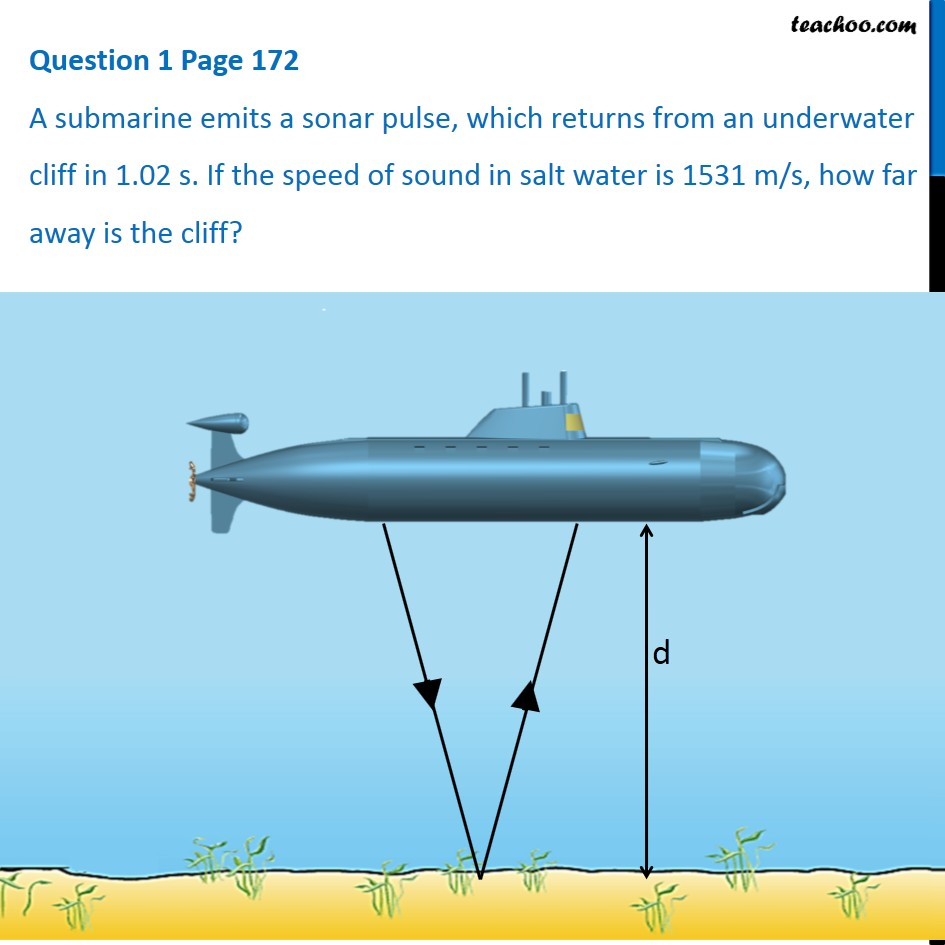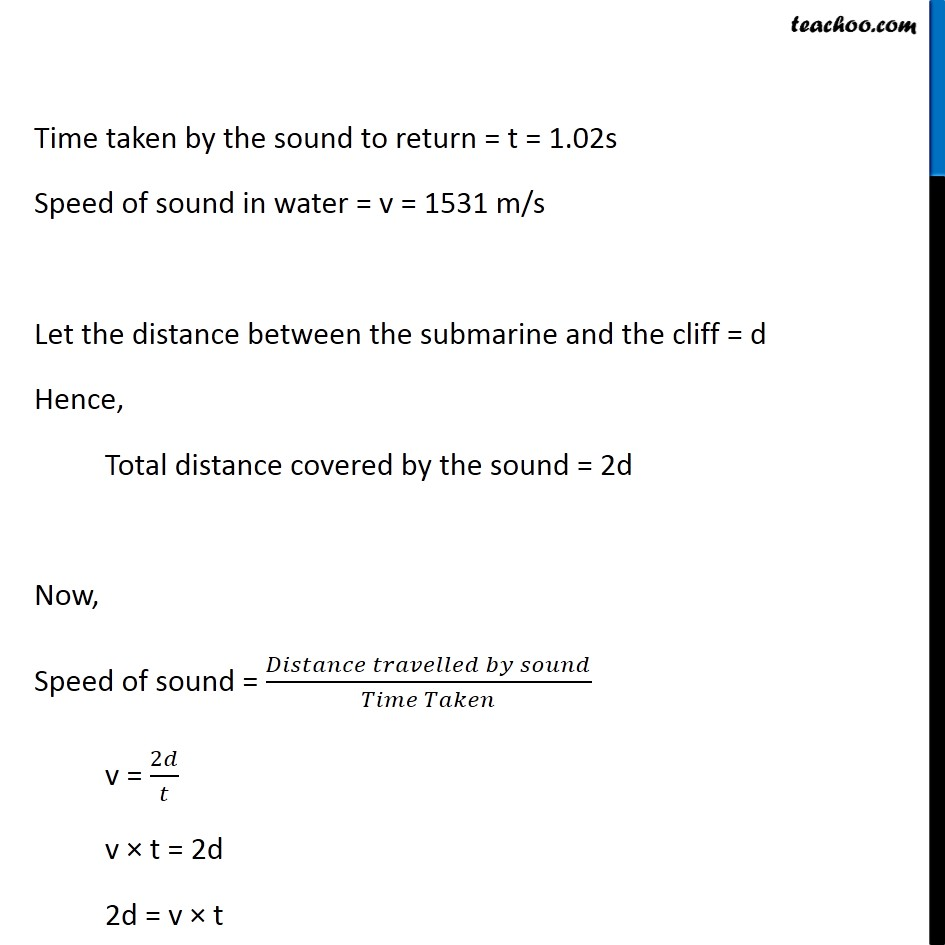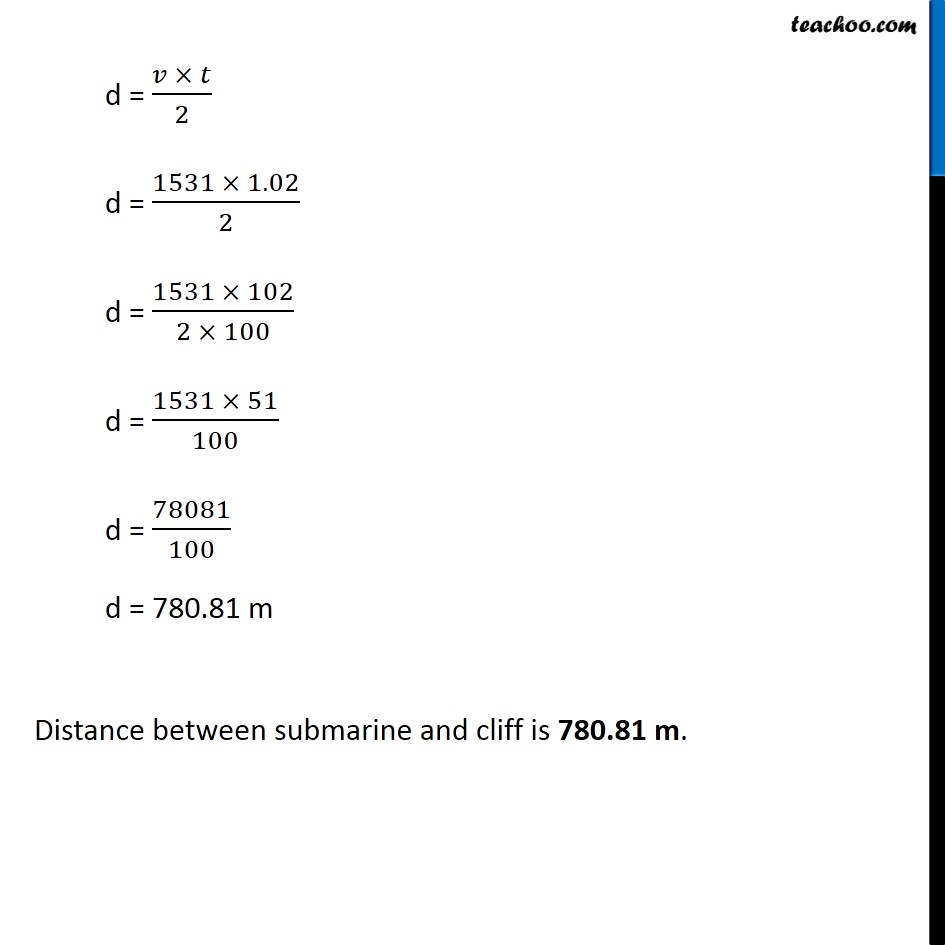Questions from inside the book

Class 9
Chapter 12 Class 9 - SoundLearn in your speed, with individual attention - Teachoo Maths 1-on-1 Class

### Transcript

Question 1 Page 172 A submarine emits a sonar pulse, which returns from an underwater cliff in 1.02 s. If the speed of sound in salt water is 1531 m/s, how far away is the cliff? Time taken by the sound to return = t = 1.02s Speed of sound in water = v = 1531 m/s Let the distance between the submarine and the cliff = d Hence, Total distance covered by the sound = 2d Now, Speed of sound = (𝐷𝑖𝑠𝑡𝑎𝑛𝑐𝑒 𝑡𝑟𝑎𝑣𝑒𝑙𝑙𝑒𝑑 𝑏𝑦 𝑠𝑜𝑢𝑛𝑑)/(𝑇𝑖𝑚𝑒 𝑇𝑎𝑘𝑒𝑛) v = 2𝑑/𝑡 v × t = 2d 2d = v × t d = (𝑣 × 𝑡)/2 d = (1531 × 1.02)/2 d = (1531 × 102)/(2 × 100) d = (1531 × 51)/100 d = 78081/100 d = 780.81 m Distance between submarine and cliff is 780.81 m.